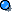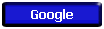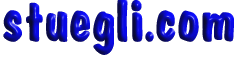#Chapter 8 - Work, Power, and Energy While most texts begin with work and power  to introduce energy, we will start out with energy on a conceptual level and use it as the basis for explaining the other concepts. Energy and momentum are all-important observables that are used in mechanics to describe the state of an object (physical body). As we already have learned Momentum is the quantity of motion of the object. Momentum is an observable that is a unique and distinct vector number that depends on mass and velocity. Energy differs from momentum in many ways. For simplicity, we will not go into all of the differences right now except for the fact that energy is a scalar measure. Energy can be most easily understood as the capacity of an object to produce changes in the state of another object. IF energy  is a capacity, then what happens when this object actually does interact with another object and cause changes to its state? Does all of the energy  from the first object get used up? does it retain some? This concept no flows naturally into the next concept, work. Work  is the amount of energy used to produce changes in the state of another object. Something can have a great deal of energy, but if it has not used it to do something, it has done no work. Finally, does it matter how fast the energy is used up while doing this work?  or better yet, is there a limit to how fast  the energy can do this work? The answer to the previous question is yes, and YES! From a practical point of view (i.e. an engineer's), an object's capacity (energy) to do work  is important, but the speed at which it can do the work is even more crucial. This  power can be simply explained. Power is how fast the work is done. In mechanics we are primarily concerned with two types of energy; kinetic  and potential. Kinetic Energy is the energy object has soley due to its motion. All objects (with mass) have kinetic energy if they are in motion. An object that has no relative motion with respect to another has no kinetic energy with which to do work on that other object. Example: Think about the shuttle astronaut space walking on a satellite repair mission. Potential Energy is the energy an object has due to its position. This energy is again a relative quantity. The position in question is, in most cases, the relative distance in elevation.  In other words, the vertical distance is the only important factor because it is in the same direction as the force (gravity) that is producing the potential energy. When thinking about potential energy  always remember that the distance, or position, is normally a difference, or delta, between two positions. In most cases you will encounter this is difference in height or elevation. (Energy has many types including nuclear, electrical, chemical, thermal, solar(radiation) and magnetic however, in reality, these are all forms of kinetic or positional energy if considered on an atomic or molecular scale. For more explanation info try http://www.ftexploring.com/energy/enrg-types.htm ) EXAMPLES - PHYZX in the REEL WURLDCopyright © 2005 -  S. B. EglI